# Mutually Inclusive Events: Definition, Examples, and Word Problems

• Author:
• Updated date:

Ray is a licensed engineer in the Philippines. He loves to write about mathematics and civil engineering.

## Mutually Inclusive Definition

Mutually inclusive events allow both events to happen at the same time or occur in a single trial. It is applied to things that must occur together, imposed by rule or natural law. Mutually inclusive events mean that two events cannot occur independently.

To fully visualize the concept of mutually inclusive events in probability, look at the Venn diagram below. As you can observe, there would be at least some overlap in the Venn diagram. The Venn diagram shows an intersection of the two circles, which suggests that C is the space or item in the two given events.

The concept of mutually inclusive events suggests that you cannot have one event without the other. That is, if X is true, then Y is true.

Mutually exclusive events do not occur at the same time. There are, however, events that can occur at the same time. The sample space for one spin on the spinner shown in the figure is S = {1, 2, 3, 4, 5, 6, 7, 8}. What is the probability of spinning an even number or a number less than 5?

These two events can be represented by the sets A = {2, 4, 6, 8} and B = {1, 2, 3, 4}. They are not mutually exclusive, as shown in the figure. If we add the probabilities of event A and event B, we will count the intersection of these events twice. If we subtract P(A ∩ B) from P(A) and P(B), we will eliminate this problem, as shown below.

P(A or B) = P(A) + P(B) - P(A ∩ B)

P(A or B) = 4/8 + 4/8 - 2/8

P(A or B) = ¾

There could be a lot more examples that show mutually inclusive events - driving your car and having a driver's license, working as an engineer and knowing how to do the math, and so much more.

Mutually Inclusive Events Theorem P (A or B) states that if A and B are events from a sample space S, then the given formula below suggests the procedure for getting the probability for mutually inclusive events.

P(A or B) = P(A) + P(B) - P(A ∩ B)

Note: Mutually inclusive events formula uses the addition rule. The use of this rule is to calculate probabilities for both mutually exclusive and inclusive events.

## Example 1: Probability of Spinning a Prime Number or an Odd Numbers

What is the probability of spinning a prime number or an odd number on a spinner numbered 1 to 8?

Scroll to Continue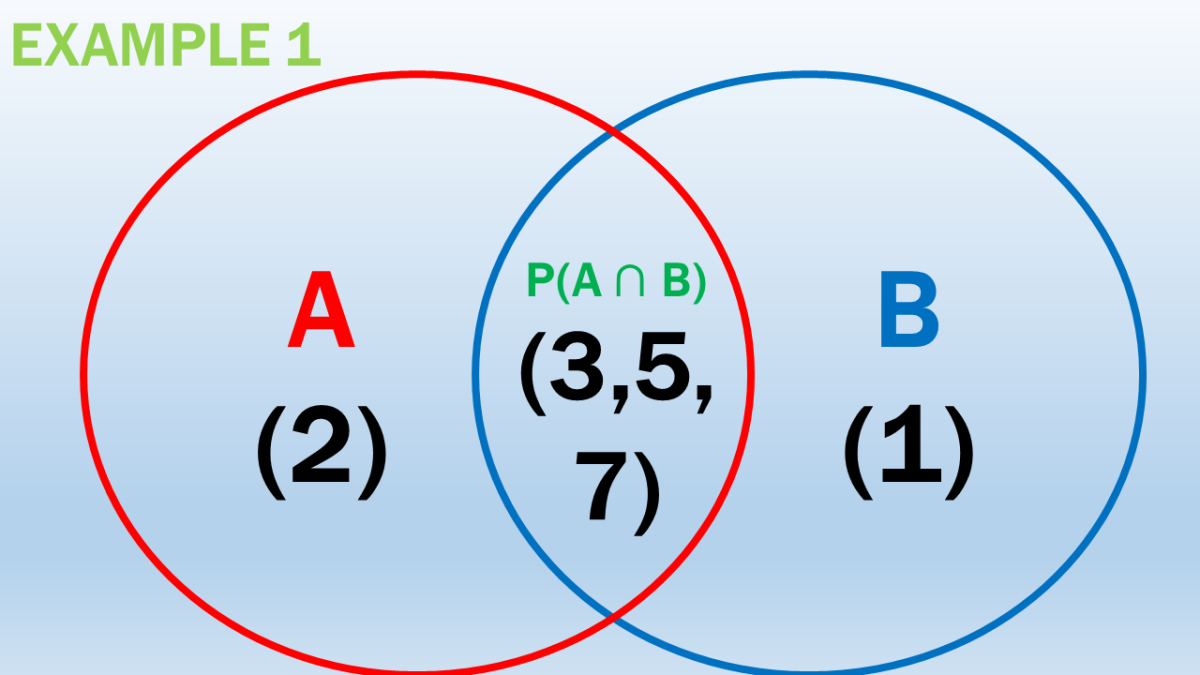Probability of Spinning a Prime Number or an Odd Numbers Using the Mutually Inclusive Events Formula

Solution

For S = {1, 2, 3, 4, 5, 6, 7, 8}, apply the theorem for mutually inclusive events.

Let A be the event of spinning a prime number.

A = {2, 3, 5, 7}

P(A) = 4/8

Let B be the event of spinning an odd number.

B = {1, 3, 5, 7}

P(B) = 4/8

For (A ∩ B) = {3, 5, 7}

P (A ∩ B) = 3/8

P(A or B) = P(A) + P(B) - P(A ∩ B)

P(A or B) = 4/8 + 4/8 - 3/8

P(A or B) = ⅝

The probability of spinning a prime number or an odd number on a spinner numbered 1 to 8 is ⅝.

## Example 2: Probability of Getting a Number Less Than 4 or 2

For numbers, one to nine, get the probability of getting a number less than 4 or 2?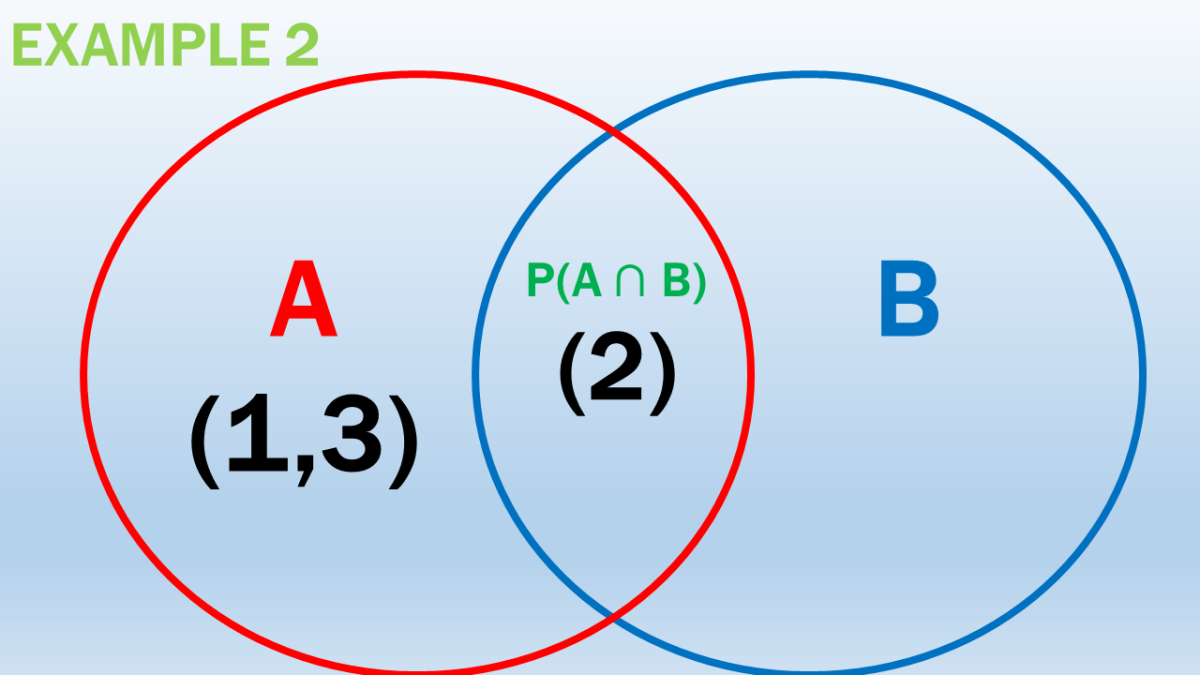Mutually Inclusive Events Probability Example in Getting a Number Less Than 4 or 2

Solution

For this problem, there could be two possible outcomes. Either you get a number less than four, and you get a number 2. For S = {1, 2, 3, 4, 5, 6, 7, 8, 9}, apply the theorem for mutually inclusive events.

Let A be the set of numbers less than 4.

A = {1, 2, 3}

P(A) = 3/9

Let B be number two.

B = {2}

P(B) = 1/9

For (A ∩ B) = {2}

P (A ∩ B) = 1/9

P(A or B) = P(A) + P(B) - P(A ∩ B)

P(A or B) = 3/9 + 1/9 - 1/9

P(A or B) = ⅓ or 33%

The probability of getting a number less than 4 or 2 is 33% or ⅓.

## Example 3: Probability of Picking an Odd Number or a Number Less Than Three

A card is drawn randomly from nine cards labelled 1 through 10. Find the probability of picking an odd number or a number less than three.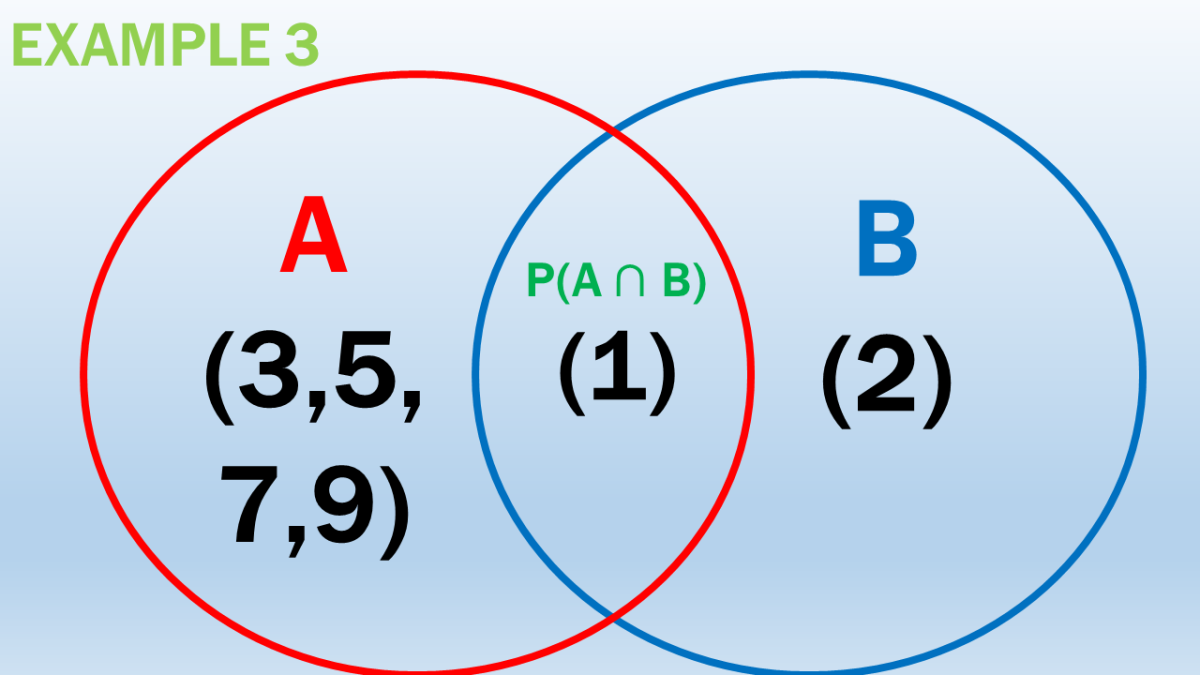Probability of Picking an Odd Number or a Number Less Than Three

Solution

Let A be the odd numbers from one to ten.

A = {1, 3, 5, 7, 9}

P(A) = 5/10

P(A) = 1/2

Let B be the numbers less than three.

B = {1, 2}

P(B) = 2/10

P(B) = 1/5

For (A ∩ B) = {1}

P (A ∩ B) = 1/10

P(A or B) = P(A) + P(B) - P(A ∩ B)

P(A or B) = 1/2 + 2/10 - 1/10

P(A or B) = ⅗ or 60%

The probability of picking an odd number or a number less than three is ⅗.

## Example 4: Probability of Getting a Queen or a Heart

A card is drawn randomly from a standard deck of cards. What is the probability of getting a queen or a heart?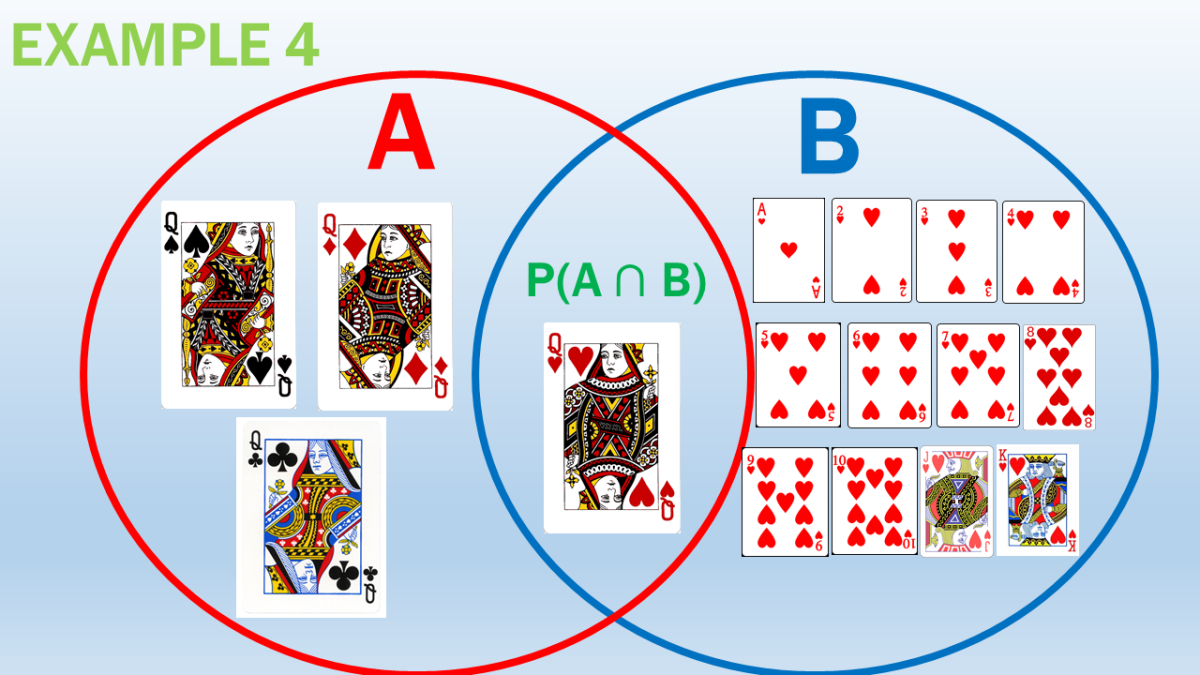Mutually Inclusive Events Example in Getting the Probability of Getting a Queen or a Heart

Solution

This problem falls under mutually inclusive events since it is possible to get a heart and a queen when choosing a card from the given deck of cards. The intersection of the two events would be the queen of hearts.

Let A be the number of queens in a standard deck of cards.

A = {queen of hearts, queen of spades, queen of clubs, queen of diamonds }

P(A) = 4/52

Let B be the hearts in the standard deck of cards.

B = {A, 2, 3, 4, 5, 6, 7, 8, 9, 10, jacks of hearts, queen of hearts, king of hearts}

P(B) = 13/52

For (A ∩ B) = {queen of hearts}

P (A ∩ B) = 1/52

P(A or B) = P(A) + P(B) - P(A ∩ B)

P(A or B) = 4/52 + 13/52 - 1/52

P(A or B) = 4/13 or 30.77%

The probability of getting a queen or a heart is 4/13 or 30.77%.

## Example 5: Probability of Getting a Club or a Ten

A card is drawn randomly from a standard deck of cards. What is the probability of getting a club or a ten?

Solution

Use the mutually inclusive event formula since it is possible to get a club and a ten when choosing a card from the given deck of cards. The intersection of the two events would be the ten of clubs.

Let A be the number of clubs in a standard deck of cards.

A = {A, 2, 3, 4, 5, 6, 7, 8, 9, 10, jacks of clubs, queen of clubs, king of clubs}

P(A) = 13/52

Let B be the number of ten cards in the standard deck of cards.

B = {ten of hearts, ten of clubs, ten of spades, ten of diamonds}

P(B) = 4/52

For (A ∩ B) = {ten of clubs}

P (A ∩ B) = 1/52

P(A or B) = P(A) + P(B) - P(A ∩ B)

P(A or B) = 13/52 + 4/52 - 1/52

P(A or B) = 4/13 or 30.77%

The probability of getting a club or a ten is 4/13 or 30.77%.

## Example 6: Dice Probability Problem

Two fair dice are rolled on the board. What is the probability of getting a sum less than seven or a sum less than 5?

Solution

The better way to illustrate the solution to this problem is by tabulating the results. Recall that dice is in a cube size and consists of 6 sides. There are a total of 36 outcomes if you roll two dice.

The probability of getting a sum less than seven or a sum less than 5.

+123456

1

2

3

4

5

6

7

2

3

4

5

6

7

8

3

4

5

6

7

8

9

4

5

6

7

8

9

10

5

6

7

8

9

10

11

6

7

8

9

10

11

12

Let A be the probability of obtaining a sum less than 7.

A = {2, 3, 4, 5, 6, 3, 4, 5, 6, 4, 5, 6, 5, 6, 6}

P(A) = 15/36

Let B be the probability of obtaining a sum less than 5.

B = {2, 3, 4, 3, 4, 4}

P(B) = 6/36

Notice that there are six elements in common, and therefore need to account for the double-counting using the mutually inclusive event's formula.

For (A ∩ B) = {2, 3, 4, 3, 4, 4}

P (A ∩ B) = 1/6

P(A or B) = P(A) + P(B) - P(A ∩ B)

P(A or B) = 15/36 + 6/36 - 1/6

P(A or B) = 5/12

The probability of getting a sum less than seven or a sum less than 5 is 5/12 or 41.6%.

## Example 7: Probability of a Four or an Even Number

A number cube is tossed on the board. What is the probability that it will be a four or an even number?

Solution

There are two possible outcomes. Either you get a number four or an even number. There is only one mutually inclusive outcome, the favourable outcome, which is 2.

Let A be the probability of obtaining a four.

A = {4}

P(A) = 1/6

Let B be the probability of obtaining an even number.

B = {2,4, 6}

P(B) = 3/6

P(B) = 1/2

1/6

For (A ∩ B) = {4}

P (A ∩ B) = 1/6

P(A or B) = P(A) + P(B) - P(A ∩ B)

P(A or B) = ⅙ + ½ - 1/6

P(A or B) = ½ or 50%

The probability that it will be a four or an even number is ½ or 50%.

## Example 8: Probability of an Eight or a Red Card From the Deck of Cards

A great magician in a park says he will throw a standard deck of cards in the air and pierce an eight or a red card (either hearts or diamonds). What is the probability of one of those events occurring?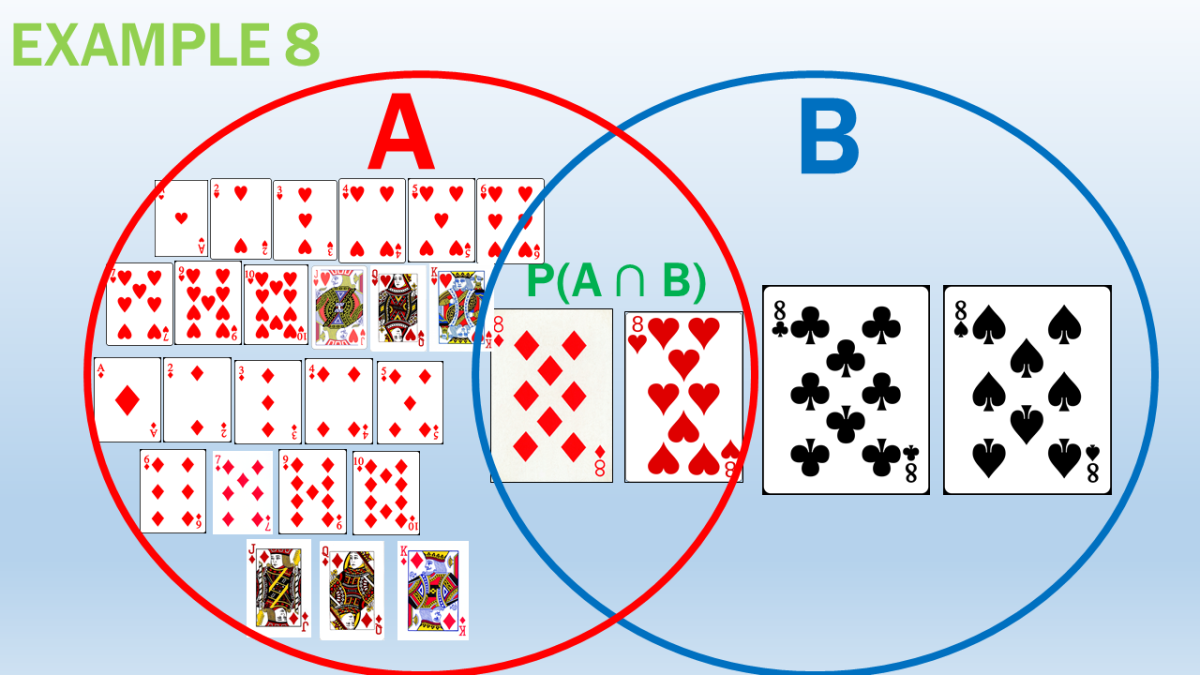Mutually Inclusive Events Problem in Getting the Probability of an Eight or a Red Card From the Deck of Cards

Solution

Let A be the probability of piercing a red card from the deck of cards. Take note that H and D stand for hearts and diamonds, respectively.

A = {H1, H2, H3, H4, H5, H6, H7, H8, H9, H10, HJ, HQ, HK, D1, D2, D3, D4, D5, D6, D7, D8, D9, D10, DJ, DQ, DK}

P(A) = 26/52

Let B be the probability of piercing an 8.

B = {8 clubs, 8 spades, 8 diamonds, 8 hearts}

P(B) = 4/52

The mutually inclusive events present in the situation are eight hearts and eight diamonds.

For (A ∩ B) = {8 hearts, 8 diamonds}

P (A ∩ B) = 2/52

P(A or B) = P(A) + P(B) - P(A ∩ B)

P(A or B) = 26/52 + 4/52 - 2/52

P(A or B) = 7/13 or 53.85%

The probability of piercing an eight or a red card (either hearts or diamonds) is 7/13 or 53.85%.

## Example 9: Probability of Getting a Jack or a Spade

If a single card is selected from a deck, find the probability that the card is either a jack or a spade.

Solution

This problem falls under mutually inclusive events since it is possible to get a jack and a spade when choosing a card from the given deck of cards. The intersection of the two events would be the jack of spades.

Let A be the event that the card is a jack.

A = {jack of hearts, jack of spades, jack of clubs, jack of diamonds}

P(A) = 4/52

Let B be the event that it is a spade from the standard deck of cards.

B = {A, 2, 3, 4, 5, 6, 7, 8, 9, 10, jacks of spades, queen of spades, king of spades}

P(B) = 13/52

For (A ∩ B) = {jack of spades}

P (A ∩ B) = 1/52

P(A or B) = P(A) + P(B) - P(A ∩ B)

P(A or B) = 4/52 + 13/52 - 1/52

P(A or B) = 4/13 or 30.77%